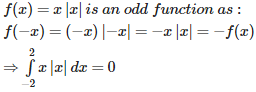# Integrals - Online Test

Q1. The function  is
Explaination / Solution: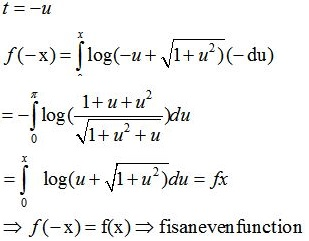Q2. t dt is equal to
Explaination / Solution:

=[sint]π/2π/2=sinπ2sin(π2)=1+1=2
Q3. dx is equal to
Explaination / Solution: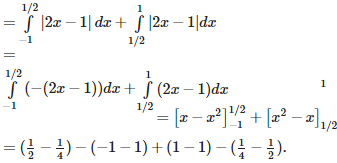Q4.  is equal to
Explaination / Solution: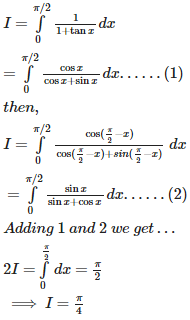Q5.  is equal to
Explaination / Solution: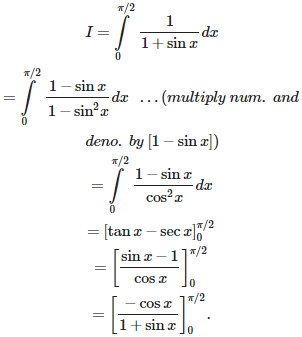Q6. dx is equal to
Explaination / Solution: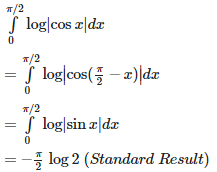Q7.  is equal to
Explaination / Solution:

Note that  is an odd function, therefore,

Q8.  (tan x) dx is equal to
Explaination / Solution: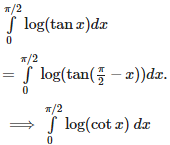Q9. (cot x) dx is equal to
Explaination / Solution: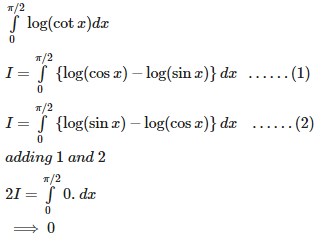Q10. is equal to
Explaination / Solution: